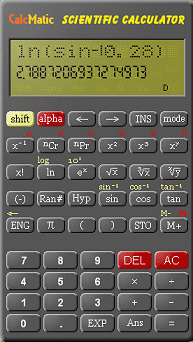Search IntMath
Close

# Free desktop scientific calculator from Yahoo!

By Murray Bourne, 23 Dec 2006

UPDATE: Yahoo Widgets are no more, unfortunately, so these tools are no longer available.

I rarely use a (physical) calculator these days. Most of the time I use Scientific Notebook when doing any mathematics problem solving because I usually want to graph my result and/or save what I have done for publication on Interactive Mathematics.

If you have need for a calculator, and a computer-based one will suffice, then you could try the Yahoo widget called CalcMatic.Then go to the CalcMatic page and download it (it's very small). It looks like this:This calculator is Javascript-based (like all the Yahoo widgets), so you should expect some mathematical anomalies at times. (See some examples in the discussion on the download page). Computers have trouble handling the conversion from base 10 (our ordinary number system) to base 8 and base 16 (used by computers, an extension of the binary system of 0s and 1s) and then back again. But on the whole, CalcMatic is not bad for a free calculator.

### Comment Preview

HTML: You can use simple tags like <b>, <a href="...">, etc.

To enter math, you can can either:

1. Use simple calculator-like input in the following format (surround your math in backticks, or qq on tablet or phone):
a^2 = sqrt(b^2 + c^2)
(See more on ASCIIMath syntax); or
2. Use simple LaTeX in the following format. Surround your math with $$ and $$.
$$\int g dx = \sqrt{\frac{a}{b}}$$
(This is standard simple LaTeX.)

NOTE: You can mix both types of math entry in your comment.